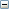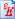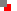﻿ DrawingLine(X1,Y1,X2,Y2:number);
 ProcedureEscapeE only. LINE procedure draws a line from X1,Y1 to X2,Y2 using the the current PEN and COLOR, see below.Line exampleColor(Red,Green,Blue: number); Colour(Red,Green,Blue: number);
 ProcedureEscapeE only. COLOR procedure (or the alternative spelling COLOUR) specifies  a color for TEXT and LINE drawing. RED, GREEN and BLUE may take values >=0, <=255 in decimal or >=\$00, <=\$FF in hexadecimal.COLOR(255,128,0) would be orange. (Equivalent to COLOR(\$FF,\$80,\$00) in Hex.)Rotate example, Line example. See also BRUSH procedure below.Brush(Red,Green,Blue: number);
 ProcedureEscapeE only. BRUSH specifies the color for filled regions; see BOX and BUTTON procedures below. RED, GREEN and BLUE may take values >=0, <=255 in decimal or >=\$00, <=\$FF in hexadecimal.BRUSH(0,255,255) would be cyan. (Equivalent to BRUSH(\$00,\$FF,\$FF) in Hex.) See also COLOR and FRAME procedures.Pen(Width:number);
 ProcedureEscapeE only. PEN specifies the pen's WIDTH in pixels (at 600dpi) for LINE drawing.Table example: Variable dataBox(X,Y,Dx,Dy:number); Box(TH:tableHandle);
 ProceduresEscapeE only. BOX fills an area DX wide by DY high at position X,Y (in pixels at 600dpi) using the color specified by BRUSH. The BOX function optionally takes a tableHandle TH (see TAB function). See also the FRAME and BUTTON functions.Button(X,Y,Dx,Dy:number); Button(TH:tableHandle);
 ProceduresEscapeE only. BUTTON draws a filled button shape (lozenge) in the selected BRUSH color. The BUTTON function optionally takes a tableHandle TH (see TAB function). See also the FRAME and BOX functions.Circle(X,Y,R[,Section]:number);
 ProcedureEscapeE only. CIRCLE draws a complete circle or a SECTION of a circle, radius R centered at position X,Y, (measured in pixels at 600dpi) using the current PEN and COLOR. If SECTION parameter is omitted a complete circle is drawn. section=0 draws a complete circle (default) section=1 draws the top right quadrant section=2 draws the bottom right quadrant section=3 draws the bottom left quadrant section=4 draws the top left quadrantRotate example, Line example.Curve(L:list);
 ProcedureEscapeE only. CURVE displays a Bezier curve. L is a list of point-coordinate pairs, consisting of a start point pair (x,y) followed groups of three coordinate pairs. (Specified in  pixels at 600dpi.)L:= [750, 1350, 150,750,   750,150,   1350,750, 1950,1350, 2550,1350, 3150,750, ... See rs2 demo files and choose poly.rs2PolyLine(L:list);
 ProcedureEscapeE only. Displays a POLYLINE linking all points specified by lists of coordinates in list L. See rs2 demo files and choose poly.rs2Frame(X,Y,Dx,Dy:number); Frame(TH:tHandle);
 ProcedureEscapeE only. FRAME draws a rectangle DX wide by DY high at X,Y (measured in pixels at 600dpi) using the selected PEN and COLOR. An overload of this function takes a Table Handle (see TAB function): a filled rectangle is drawn using the BOX function.